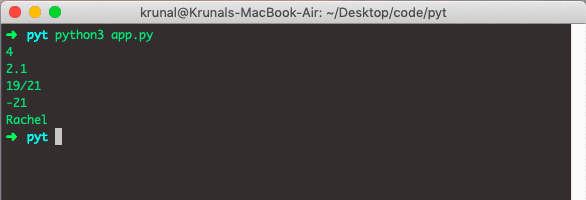# Python Mode: The Complete Guide

## Python mode

Python mode() is a built-in function in a statistics module that applies to nominal (non-numeric) data. The mode() is used to locate the central tendency of numeric or nominal data.

Python statistics module has a considerable number of functions to work with very large data sets. The mode() function is one of such methods. The mode is a value at which the data is most likely to be sampled.

If you are looking for the most occurring number in the list, array, or tuple then the Python mode() function is the answer you are looking for.

The mode is the statistical term that refers to the most frequently occurring number found in a set of numbers. The mode is detected by collecting and organizing data to count the frequency of each result.

In Python, we use the Statistics module to calculate the mode. See the following example.

```# app.py

import statistics

listA = [19, 21, 46, 19, 18, 19]
print(statistics.mode(listA))
```

In the above code, number 19 is frequently appearing. So that is our mode.

See the below output.Now, let’s see the unique item list.

```# app.py

import statistics

listUnique = [19, 21, 18, 30, 46]
print(statistics.mode(listUnique))```

The above list has unique elements inside the list. So mode does not work here. Instead, it will give us an error.

See the below output.## Calculate Mode of Tuple in Python

To calculate the mode of the tuple, just pass the tuple as a parameter to the mode() function and it will return the mode of data.

Let’s define a tuple and calculate the mode of the tuple.

```# app.py

import statistics

data = (21, 19, 18, 46, 30, 18, 19, 21, 18)
print(statistics.mode(data))
```

Let’s see the output.## More Examples

Let’s add more examples to the app.py file.

```# app.py

import statistics
from fractions import Fraction as fr

data1 = (1, 2, 2, 3, 3, 3, 4, 4, 4, 4)
print(statistics.mode(data1))

data2 = (2.1, 1.9, 2.1, 1.8, 2.9)
print(statistics.mode(data2))

data3 = (fr(19, 21), fr(18, 21), fr(19, 21), fr(21, 46))
print(statistics.mode(data3))

data4 = (-21, -22, -21, -29, -18, -19)
print(statistics.mode(data4))

data5 = ('Emily', 'Matt', 'Ross', 'Rachel', 'Monica', 'Chandler', 'Rachel')
print(statistics.mode(data5))```

See the output below.It will work with Strings as well, as we have defined the list of strings in the last example.

That’s it for this tutorial.

This site uses Akismet to reduce spam. Learn how your comment data is processed.